# LESSON 10 NORMAL DISTRIBUTION Outline Normal distribution Area

• Slides: 23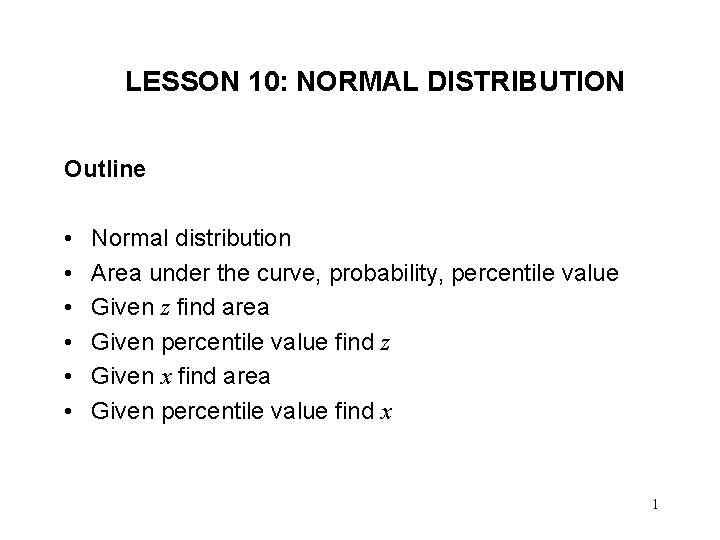LESSON 10: NORMAL DISTRIBUTION Outline • • • Normal distribution Area under the curve, probability, percentile value Given z find area Given percentile value find z Given x find area Given percentile value find x 1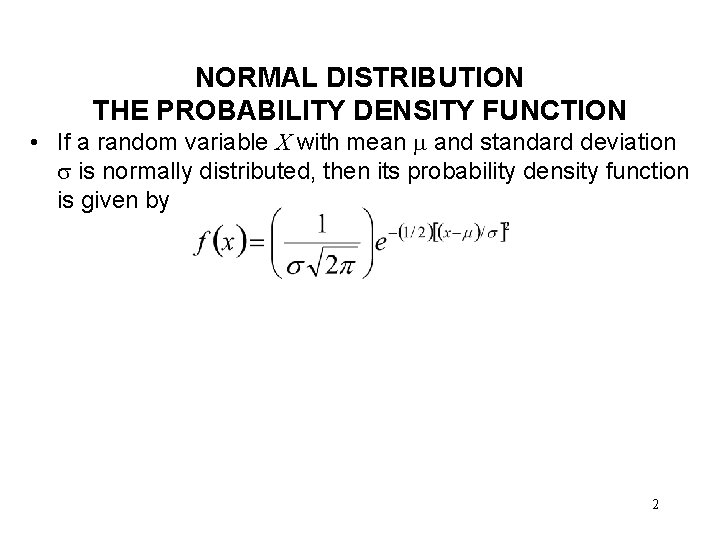NORMAL DISTRIBUTION THE PROBABILITY DENSITY FUNCTION • If a random variable X with mean and standard deviation is normally distributed, then its probability density function is given by 2NORMAL DISTRIBUTION THE PROBABILITY DENSITY FUNCTION f (x ) Mean, =50 SD, =10 0. 0500 f (x ) 0. 0400 0. 0300 Area between the vertical lines = P (40 X 60) Area under the curve = 1. 00 0. 0200 0. 0100 0. 0000 0 20 40 60 x - VALUES 80 100 3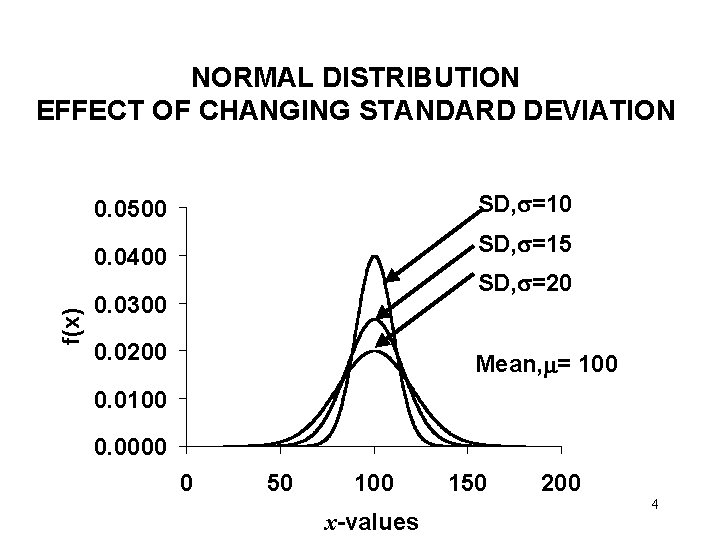NORMAL DISTRIBUTION EFFECT OF CHANGING STANDARD DEVIATION SD, =10 0. 0500 SD, =15 f(x) 0. 0400 SD, =20 0. 0300 0. 0200 Mean, = 100 0. 0000 0 50 100 x-values 150 200 4NORMAL DISTRIBUTION EFFECT OF CHANGING MEAN SD, =10 5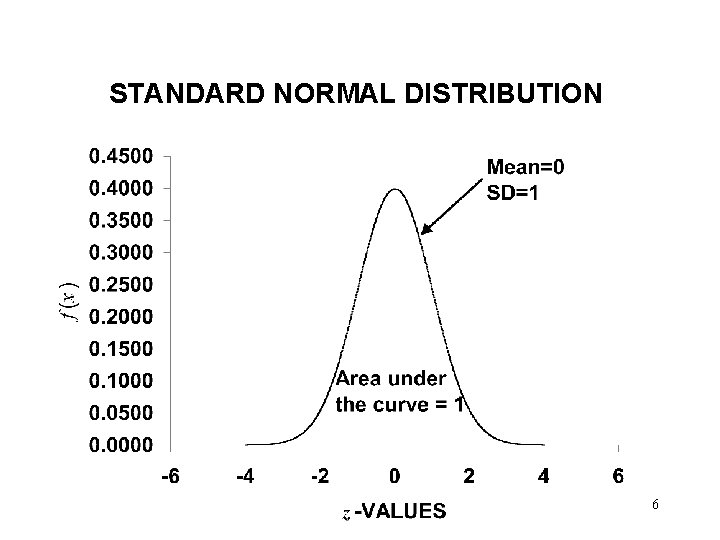STANDARD NORMAL DISTRIBUTION 6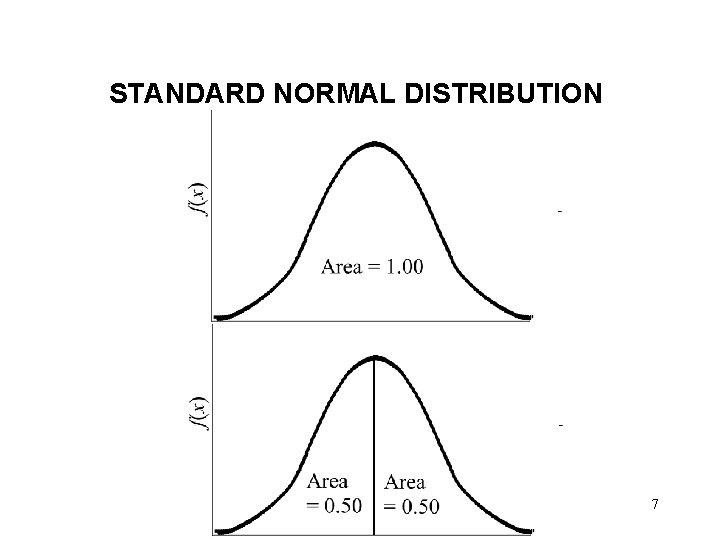STANDARD NORMAL DISTRIBUTION 7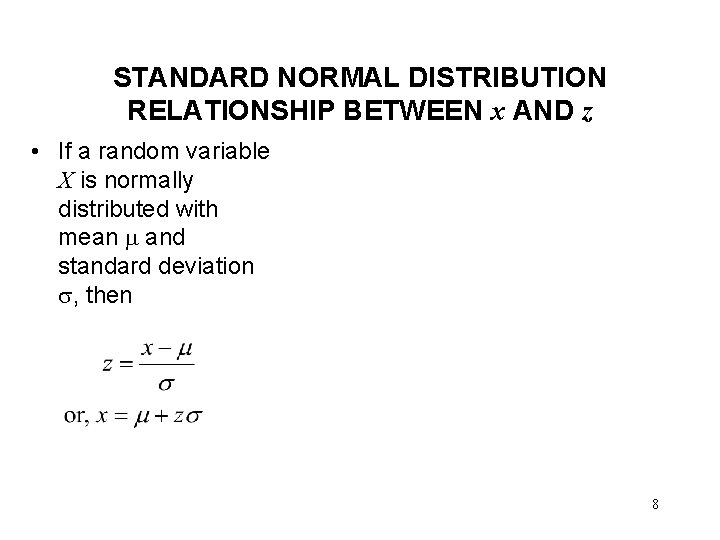STANDARD NORMAL DISTRIBUTION RELATIONSHIP BETWEEN x AND z • If a random variable X is normally distributed with mean and standard deviation , then 8STANDARD NORMAL DISTRIBUTION TABLE, z-VALUES, AREA AND PROBABILITY Example 1. 1: Table D, Appendix A, pp. 536 -537 shows the area under the curve from Z=-∞ to some z value. For example, the area from Z=-∞ to Z=1. 3+. 04=1. 34 is 0. 9099. So, 9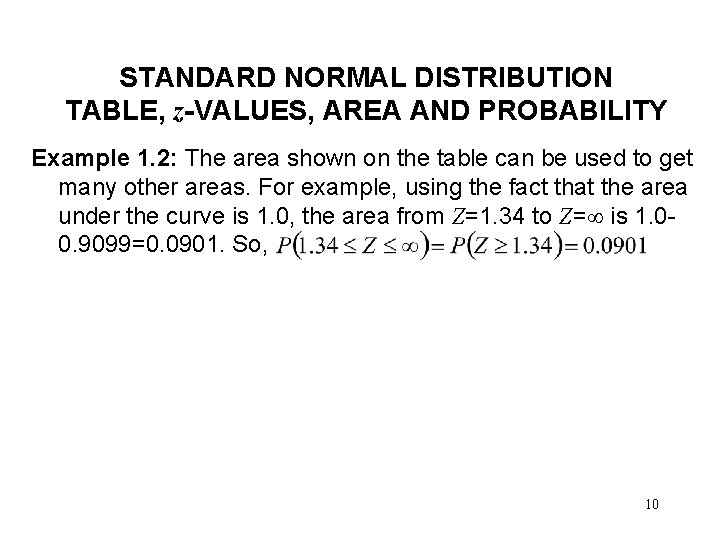STANDARD NORMAL DISTRIBUTION TABLE, z-VALUES, AREA AND PROBABILITY Example 1. 2: The area shown on the table can be used to get many other areas. For example, using the fact that the area under the curve is 1. 0, the area from Z=1. 34 to Z= is 1. 00. 9099=0. 0901. So, 10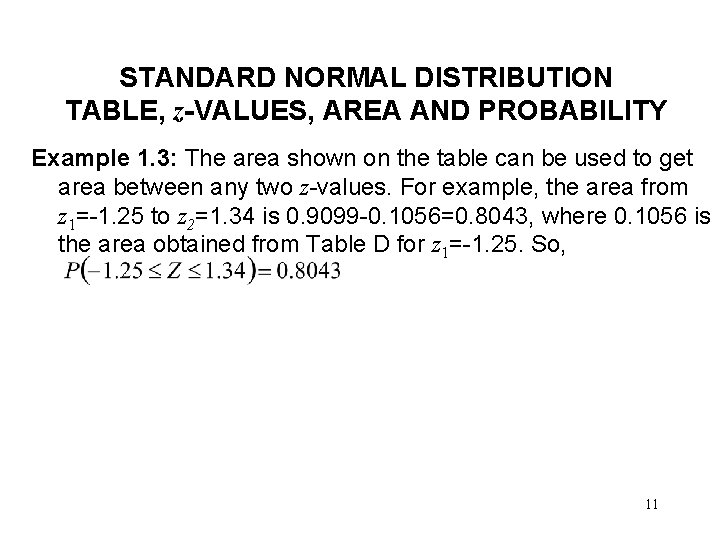STANDARD NORMAL DISTRIBUTION TABLE, z-VALUES, AREA AND PROBABILITY Example 1. 3: The area shown on the table can be used to get area between any two z-values. For example, the area from z 1=-1. 25 to z 2=1. 34 is 0. 9099 -0. 1056=0. 8043, where 0. 1056 is the area obtained from Table D for z 1=-1. 25. So, 11STANDARD NORMAL DISTRIBUTION GIVEN z, FIND PROBABILITY Example 2: Find the following: 12STANDARD NORMAL DISTRIBUTION GIVEN z, FIND PROBABILITY : EXCEL • Excel function NORMSDIST(z) provides the area under the standard normal distribution curve on the left side of z. • Example: NORMSDIST(1. 34) = 0. 9099 = To get the area on the left of Z = 1. 34, Φ(1. 34), use Excel function NORMSDIST(1. 34). Area =? z=1. 34 13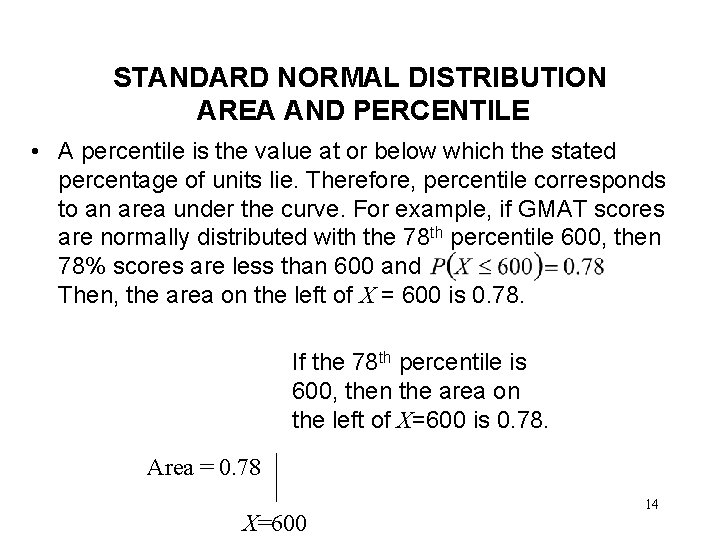STANDARD NORMAL DISTRIBUTION AREA AND PERCENTILE • A percentile is the value at or below which the stated percentage of units lie. Therefore, percentile corresponds to an area under the curve. For example, if GMAT scores are normally distributed with the 78 th percentile 600, then 78% scores are less than 600 and Then, the area on the left of X = 600 is 0. 78. If the 78 th percentile is 600, then the area on the left of X=600 is 0. 78. Area = 0. 78 X=600 14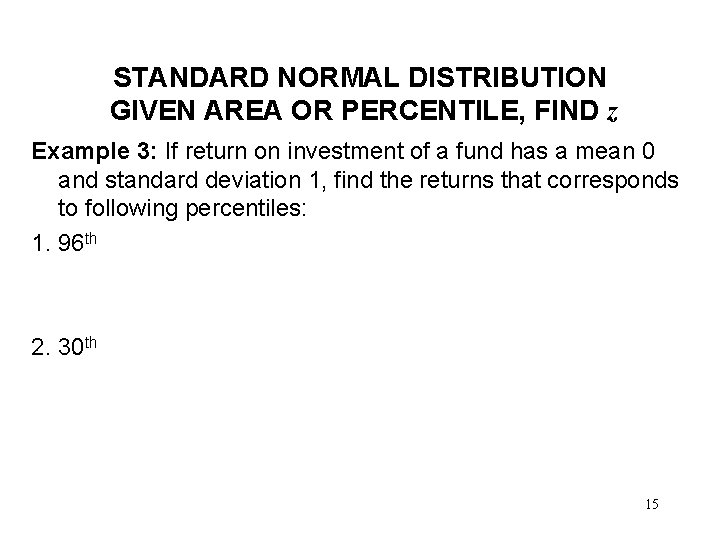STANDARD NORMAL DISTRIBUTION GIVEN AREA OR PERCENTILE, FIND z Example 3: If return on investment of a fund has a mean 0 and standard deviation 1, find the returns that corresponds to following percentiles: 1. 96 th 2. 30 th 15STANDARD NORMAL DISTRIBUTION GIVEN AREA OR PERCENTILE, FIND z : EXCEL • Excel function NORMSINV(p) provides the value of z corresponding to the 100 pth percentile. For example, NORMSINV(0. 33)=-0. 44. So, for the standard normal distribution, the 33 rd percentile is -0. 44. To get the z value for which area on the left, Φ(z) = 0. 33, use Excel function NORMSINV(0. 33). Area =0. 33 z=? 16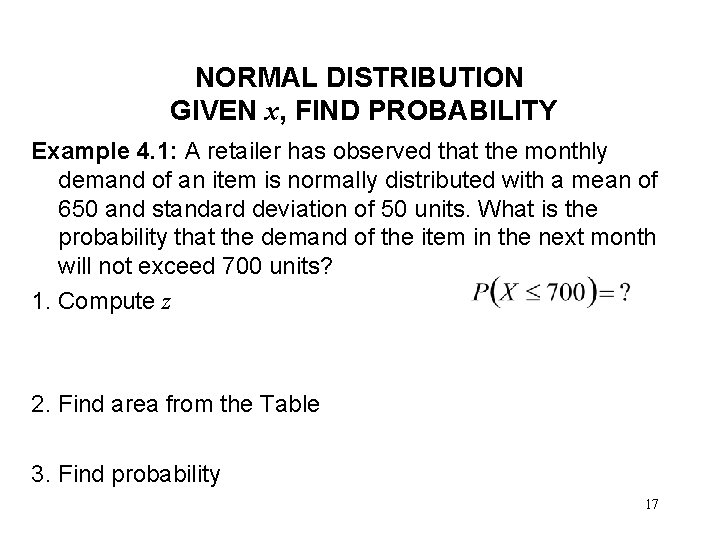NORMAL DISTRIBUTION GIVEN x, FIND PROBABILITY Example 4. 1: A retailer has observed that the monthly demand of an item is normally distributed with a mean of 650 and standard deviation of 50 units. What is the probability that the demand of the item in the next month will not exceed 700 units? 1. Compute z 2. Find area from the Table 3. Find probability 17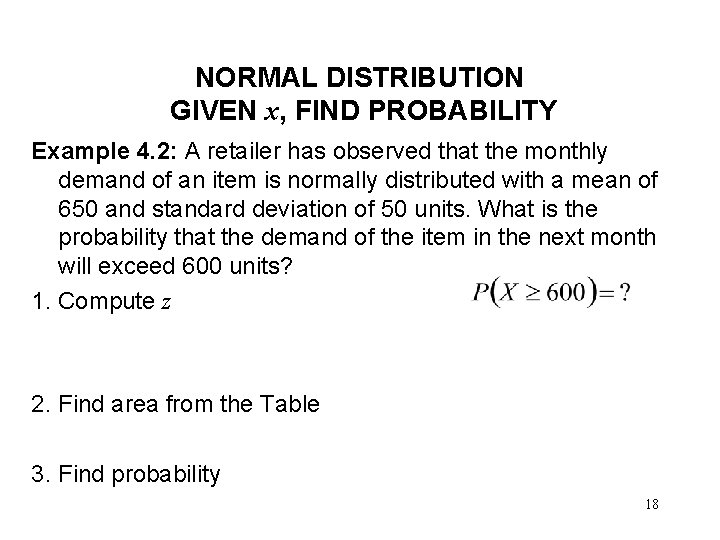NORMAL DISTRIBUTION GIVEN x, FIND PROBABILITY Example 4. 2: A retailer has observed that the monthly demand of an item is normally distributed with a mean of 650 and standard deviation of 50 units. What is the probability that the demand of the item in the next month will exceed 600 units? 1. Compute z 2. Find area from the Table 3. Find probability 18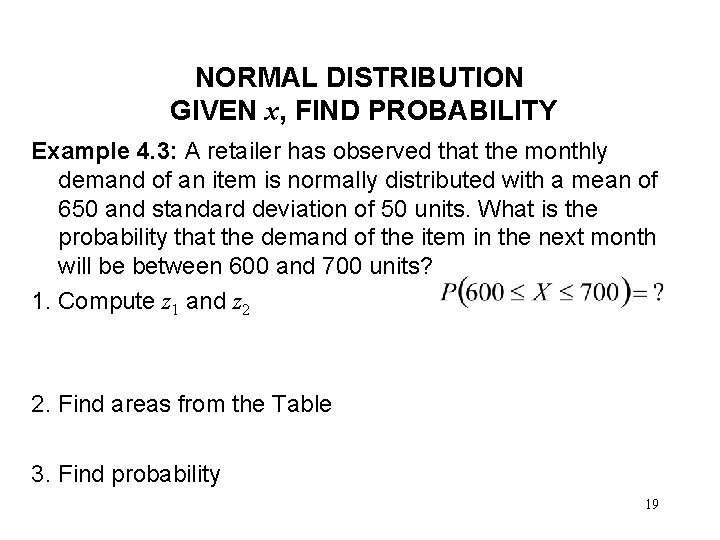NORMAL DISTRIBUTION GIVEN x, FIND PROBABILITY Example 4. 3: A retailer has observed that the monthly demand of an item is normally distributed with a mean of 650 and standard deviation of 50 units. What is the probability that the demand of the item in the next month will be between 600 and 700 units? 1. Compute z 1 and z 2 2. Find areas from the Table 3. Find probability 19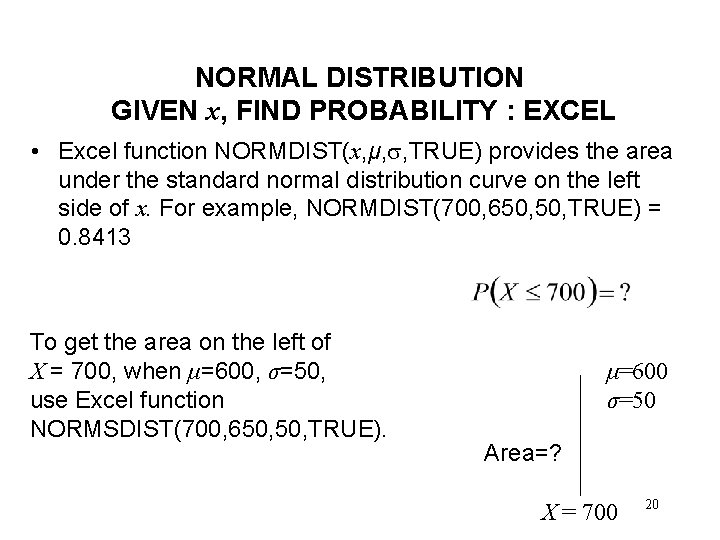NORMAL DISTRIBUTION GIVEN x, FIND PROBABILITY : EXCEL • Excel function NORMDIST(x, μ, , TRUE) provides the area under the standard normal distribution curve on the left side of x. For example, NORMDIST(700, 650, TRUE) = 0. 8413 To get the area on the left of X = 700, when μ=600, σ=50, use Excel function NORMSDIST(700, 650, TRUE). μ=600 σ=50 Area=? X = 700 20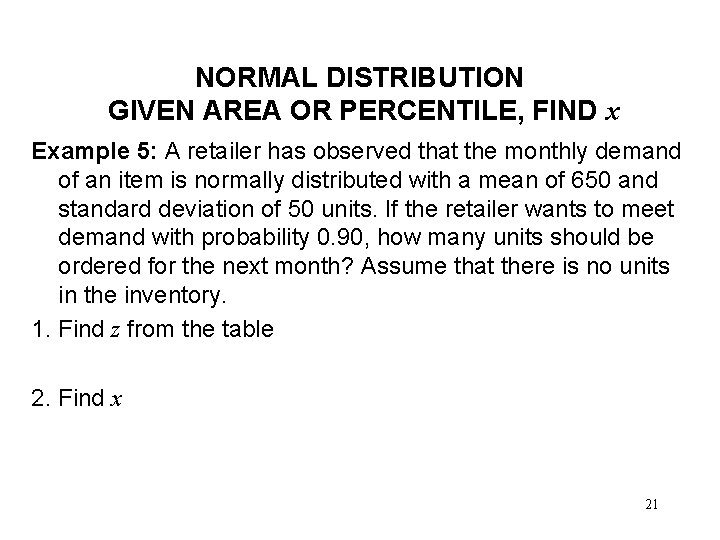NORMAL DISTRIBUTION GIVEN AREA OR PERCENTILE, FIND x Example 5: A retailer has observed that the monthly demand of an item is normally distributed with a mean of 650 and standard deviation of 50 units. If the retailer wants to meet demand with probability 0. 90, how many units should be ordered for the next month? Assume that there is no units in the inventory. 1. Find z from the table 2. Find x 21STANDARD NORMAL DISTRIBUTION GIVEN AREA OR PERCENTILE, FIND x : EXCEL • Excel function NORMINV(p, μ, ) provides 100 pth percentile when mean is μ and standard deviation . So, the function also gives that value of X for which the area on the left side of X is p. For example, NORMINV(0. 90, 650, 50) = 714. So, the 90 th percentile is 714. To get the x value for which area on the left = 0. 90, use Excel function NORMSINV(0. 90, 650, 50) if mean is 650 and standard deviation 50. μ=600 σ=50 Area = 0. 90 X=? 22READING AND EXERCISES Lesson 10 Reading: Section 7 -4, pp. 216 -225 Exercises: 7 -35, 7 -36, 7 -37 23